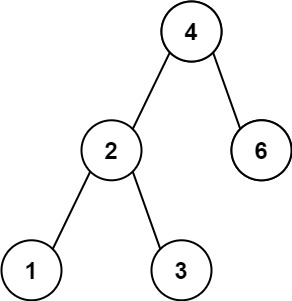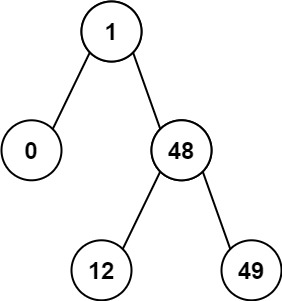# GeetCode Hub

Given the `root` of a Binary Search Tree (BST), return the minimum absolute difference between the values of any two different nodes in the tree.

Example 1:```Input: root = [4,2,6,1,3]
Output: 1
```

Example 2:```Input: root = [1,0,48,null,null,12,49]
Output: 1
```

Constraints:

• The number of nodes in the tree is in the range `[2, 104]`.
• `0 <= Node.val <= 105`

Note: This question is the same as 783: https://leetcode.com/problems/minimum-distance-between-bst-nodes/

/** * Definition for a binary tree node. * public class TreeNode { * int val; * TreeNode left; * TreeNode right; * TreeNode() {} * TreeNode(int val) { this.val = val; } * TreeNode(int val, TreeNode left, TreeNode right) { * this.val = val; * this.left = left; * this.right = right; * } * } */ class Solution { public int getMinimumDifference(TreeNode root) { } }